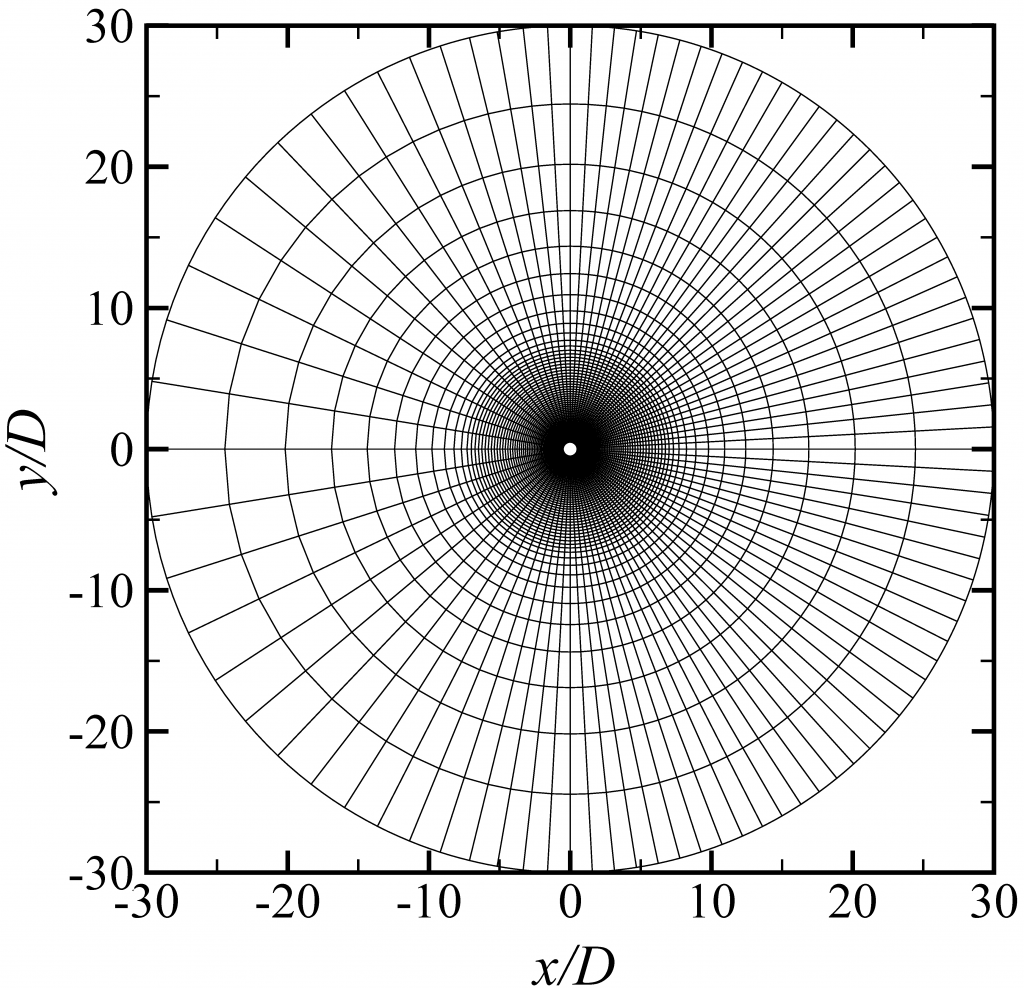# Flow past a circular cylinder: numerical convergence and computational efficiency

by Hongyi Jiang (University of Western Australia)

A steady approaching flow past a smooth circular cylinder is a popular benchmarking case for CFD. By using Nektar++, we (https://doi.org/10.1063/5.0041168) reported a systematic numerical convergence study for the case of flow past a circular cylinder at Re = 3900, which included separate examinations of (i) the spanwise domain length, (ii) the spanwise resolution via changing the number of Fourier planes, (iii) the resolution in the plane perpendicular to the spanwise direction via either h– or p-type refinement, and (iv) the spectral vanishing viscosity (SVV) coefficients. The computational cost for a satisfactory level of numerical convergence was approximately 30,000 core hours (2×24 cores on a Cray XC40 system supercomputer and for a time integration of 1000 non-dimensional time units).

The mesh is shown in the figure below.Macro-element mesh for the Nektar++ model (used together with a polynomial order of 4 and 64 Fourier planes over a spanwise domain length of 3 cylinder diameters). For the OpenFOAM model, the cell sizes were generally five times smaller in both the x- and y-directions.

We also simulated this case with OpenFOAM (v5.0), based on the conventional finite volume method (FVM) and the WALE LES model. A quantitative comparison of the computational efficiency for the OpenFOAM and Nektar++ models is meaningful when both numerical simulations reach a satisfactory level of mesh convergence. We estimated based on extrapolation of the mesh dependence results that for the OpenFOAM model the computational cost for mesh convergence was approximately one million core hours. In comparison, the Nektar++ formulation, which includes the spectral/hp element method coupled with a Fourier expansion in the spanwise direction, offers a greater computational efficiency (the computational cost was more than one order of magnitude smaller) and is therefore recommended for the numerical modelling of bluff-body flows.

Based on the Nektar++ approach, the computations were generalised to a range of Re = 400 – 3900. The Nektar++ results agreed well with the experimental results over the range of Re investigated, which demonstrated accuracy and robustness of the Nektar++ approach.